Chemical Reactions and Kinetics

The Mechanisms of Chemical Reactions

What happens when the first step in a multi-step reaction is not the rate-limiting step? Consider the reaction between NO and O2 to form NO2, for example.

2 NO(g) + O2(g)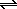2 NO2(g)

This reaction involves a two-step mechanism. The first step is a relatively fast reaction in which a pair of NO molecules combine to form a dimer, N2O2. The product of this step then undergoes a much slower reaction in which it combines with O2 to form a pair of NO2 molecules.

 Step 1: 2 NON2O2 (fast step) Step 2: N2O2 + O22 NO2 (slow step)

The net effect of these reactions is the transformation of two NO molecules and one O2 molecule into a pair of NO2 molecules.

 2 NON2O2 N2O2 + O22 NO2 _______________ 2 NO + O22 NO2

In this reaction, the second step is the rate-limiting step. No matter how fast the first step takes place, the overall reaction cannot proceed any faster than the second step in the reaction. As we have seen, the rate of any step in a reaction is directly proportional to the concentrations of the reactants consumed in that step. The rate law for the second step in this reaction is therefore proportional to the concentrations of both N2O2 and O2.

 Step 2: Rate2nd = k(N2O2)(O2)

Because the first step in the reaction is much faster, the overall rate of reaction is more or less equal to the rate of this rate-limiting step.

Rate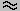k(N2O2)(O2)

This rate law is not very useful because it is difficult to measure the concentrations of intermediates, such as N2O2, that are simultaneously formed and consumed in the reaction. It would be better to have an equation that related the overall rate of reaction to the concentrations of the original reactants.

Let's take advantage of the fact that the first step in this reaction is reversible.

 Step 1: 2 NON2O2

The rate of the forward reaction in this step depends on the concentration of NO raised to the second power.

 Step 1: Rateforward = kf(NO)2

The rate of the reverse reaction depends only on the concentrations of N2O2.

 Step 1: Ratereverse = kr(N2O2)

Because the first step in this reaction is very much faster than the second, the first step should come to equilibrium. When that happens, the rate of the forward and reverse reactions for the first step are the same.

kf(NO)2 = kr(N2O2)

Let's rearrange this equation to solve for one of the terms that appears in the rate law for the second step in the reaction.

(N2O2) = (kf /kr) (NO)2

Substituting this equation into the rate law for the second step gives the following result.

Rate2nd = k (kf /kr) (NO)2(O2)

Since k, kf, and kr are all constants, they can be replaced by a single constant, k', to give the experimental rate law for this reaction described in Exercise 22.6.

RateoverallRate2nd = k'(NO)2(O2)The Relationship Between the Rate Constants and the Equilibrium Constant for a Reaction

There is a simple relationship between the equilibrium constant for a reversible reaction and the rate constants for the forward and reverse reactions if the mechanism for the reaction involves only a single step. To understand this relationship, let's turn once more to a reversible reaction that we know occurs by a one-step mechanism.

ClNO2(g) + NO(g)NO2(g) + ClNO(g)

The rate of the forward reaction is equal to a rate constant for this reaction, kf, times the concentrations of the reactants, ClNO2 and NO.

Rateforward = kf(ClNO2)(NO)

The rate of the reverse reaction is equal to a second rate constant, kr, times the concentrations of the products, NO2 and ClNO.

Ratereverse = kr(NO2)(ClNO)

This system will reach equilibrium when the rate of the forward reaction is equal to the rate of the reverse reaction.

Rateforward = Ratereverse

Substituting the rate laws for the forward and reverse reactions when the system is at equilibrium into this equation gives the following result.

kf[NO][ClNO2] = kr[ClNO][NO2]

This equation can be rearranged to give the equilibrium constant expression for the reaction.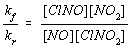Thus, the equilibrium constant for a one-step reaction is equal to the forward rate constant divided by the reverse rate constant.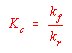Practice Problem 7:The rate constants for the forward and reverse reactions in the following equilibrium have been measured.   At 25°C, kf is 7.3 x 103 liters per mole-second and kr is 0.55 liters per mole-second.  Calculate the equilibrium constant for this reaction: ClNO2(g) + NO(g)NO2(g) + ClNO(g) Click here to check your answer to Practice Problem 7. Click here to see a solution to Practice Problem 7.Determining the Order of a Reaction from Rate of Reaction Data

The rate law for a reaction can be determined by studying what happens to the initial instantaneous rate of reaction when we start with different initial concentrations of the reactants. To show how this is done, let's determine the rate law for the decomposition of hydrogen peroxide in the presence of the iodide ion.

 2 H2O2(aq)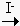2 H2O(aq) + O2(g)

Data on initial instantaneous rates of reaction for five experiments run at different initial concentrations of H2O2 and the I- ion are given in the table below.

Rate of Reaction Data for the Decomposition of H2O2 in the Presence of the I- Ion

 Initial (H2O2) (M) Initial (I-) (M) Initial Instantaneous Rate of Reaction (M/s) Trial 1: 1.0 x 10-2 2.0 x 10-3 2.3 x 107 Trial 2: 2.0 x 10-2 2.0 x 10-3 4.6 x 107 Trial 3: 3.0 x 10-2 2.0 x 10-3 6.9 x 107 Trial 4: 1.0 x 10-2 4.0 x 10-3 4.6 x 107 Trial 5: 1.0 x 10-2 6.0 x 10-3 6.9 x 107

The only difference between the first three trials is the initial concentration of H2O2. The difference between Trial 1 and Trial 2 is a two-fold increase in the initial H2O2 concentration, which leads to a two-fold increase in the initial rate of reaction.The difference between Trial 1 and Trial 3 is a three-fold increase in the initial H2O2 concentration, which produces a three-fold increase in the initial rate of reaction.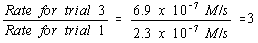The only possible conclusion is that the rate of reaction is directly proportional to the H2O2 concentration.

Experiments 1, 4, and 5 were run at the same initial concentration of H2O2 but different initial concentrations of the I- ion. When we compare Trials 1 and 4 we see that doubling the initial I- concentration leads to a twofold increase in the rate of reaction.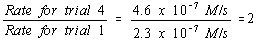Trials 1 and 5 show that tripling the initial I- concentration leads to a three-fold increase in the initial rate of reaction. We therefore conclude that the rate of the reaction is also directly proportional to the concentration of the I- ion.

The results of these experiments are consistent with a rate law for this reaction that is first-order in both H2O2 and I-.

Rate = k(H2O2)(I-)

 Practice Problem 8:Hydrogen iodide decomposes to give a mixture of hydrogen and iodine: 2 HI(g)H2(g) + I2(g) Use the following data to determine whether the decomposition of HI in the gas phase is first order or second order in hydrogen iodide.                Initial (HI) (M)         Initial Instantaneous                                                  Rate of Reaction (M/s)          Trial 1:     1.0 x 10-2                   4.0 x 10-6 Trial 2:     2.0 x 10-2                   1.6 x 10-5 Trial 3:     3.0 x 10-2                   3.6 x 10-5 Click here to check your answer to Practice Problem 8. Click here to see a solution to Practice Problem 8.The Integrated Form of First-Order and Second-Order Rate Laws

The rate law for a reaction is a useful way of probing the mechanism of a chemical reaction but it isn't very useful for predicting how much reactant remains in solution or how much product has been formed in a given amount of time. For these calculations, we use the integrated form of the rate law.

Let's start with the rate law for a reaction that is first-order in the disappearance of a single reactant, X.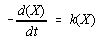When this equation is rearranged and both sides are integrated we get the following result.

 Integrated form of the first-order rate law: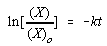In this equation, (X) is the concentration of X at any moment in time, (X)0 is the initial concentration of this reagent, k is the rate constant for the reaction, and t is the time since the reaction started.

To illustrate the power of the integrated form of the rate law for a reaction, let's use this equation to calculate how long it would take for the 14C in a piece of charcoal to decay to half of its original concentration. We will start by noting that 14C decays by first-order kinetics with a rate constant of 1.21 x 10-4 yr-1.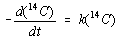The integrated form of this rate law would be written as follows.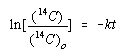We are interested in the moment when the concentration of 14C in the charcoal is half of its initial value.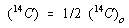Substituting this relationship into the integrated form of the rate law gives the following equation.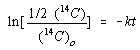We now simplify this equation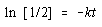and then solve for t.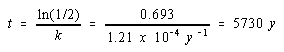It therefore takes 5730 years for half of the 14C in the sample to decay. This is called the half-life of 14C. In general, the half-life for a first-order kinetic process can be calculated from the rate constant as follows.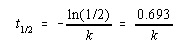Let's now turn to the rate law for a reaction that is second-order in a single reactant, X.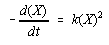The integrated form of the rate law for this reaction is written as follows.

 Integrated form of the second-order rate law: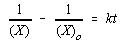Once again, (X) is the concentration of X at any moment in time, (X)0 is the initial concentration of X, k is the rate constant for the reaction, and t is the time since the reaction started.

 Practice Problem 9:Acetaldehyde, CH3CHO, decomposes by second-order kinetics with a rate constant of 0.334 M-1s-1 at 500°C.  Calculate the amount of time it would take for 80% of the acetaldehyde to decompose in a sample that has an initial concentration of 0.00750 M. Click here to check your answer to Practice Problem 9. Click here to see a solution to Practice Problem 9.

The half-life of a second-order reaction can be calculated from the integrated form of the second-order rate law.We start by asking: "How long it would take for the concentration of X to decay from its initial value, (X)0, to a value half as large?"The first step in simplifying this equation involves multiplying the top and bottom halves of the first term by 2.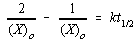Subtracting one term on the left side of this equation from the other gives the following result.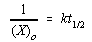We can now solve this equation for the half-life of the reaction.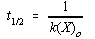There is an important difference between the equations for calculating the half-life of first order and second-order reactions. The half-life of a first-order reaction is a constant, which is proportional to the rate constant for the reaction.

 first-order reaction: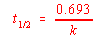The half-life for a second-order reaction is inversely proportional to both the rate constant for the reaction and the initial concentration of the reactant that is consumed in the reaction.

 second-order reaction: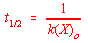Discussions of the half-life of a reaction are therefore usually confined to first-order processes.Determining the Order of a Reaction with the Integrated form of Rate Laws

The integrated form of the rate laws for first- and second-order reactions provides another way of determining the order of a reaction. We can start by assuming, for the sake of argument, that the reaction is first-order in reactant X.

Rate = k(X)

We then test this assumption by checking concentration versus time data for the reaction to see whether they fit the first-order rate law.To see how this is done, let's start by rearranging the integrated form of the first-order rate law as follows.

ln (X) - ln (X)0 = - kt

We then solve this equation for the natural logarithm of the concentration of X at any moment in time.

ln (X) = ln (X)0 - kt

This equation contains two variablesln (X) and tand two constantsln (X)0 and k. It can therefore be set up in terms of the equation for a straight line.

y = mx + b

ln (X) = -kt + ln (X)0

If the reaction is first-order in X, a plot of the natural logarithm of the concentration of X versus time will be a straight line with a slope equal to -k, as shown in the figure below.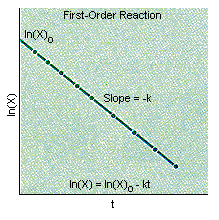If the plot of ln (X) versus time is not a straight line, the reaction can't be first-order in X. We therefore assume, for the sake of argument, that it is second-order in X.

Rate = k(X)2

We then test this assumption by checking whether the experimental data fit the integrated form of the second-order rate law.This equation contains two variables(X) and tand two constants(X)0 and k. Thus, it also can be set up in terms of the equation for a straight line.

y = mx + bIf the reaction is second-order in X, a plot of the reciprocal of the concentration of X versus time will be a straight line with a slope equal to k, as shown in the figure below.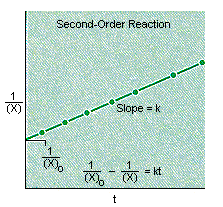Practice Problem 10:Use the experimental data found in the table at the beginning of this lesson to determine whether the reaction between phenolphthalein (PHTH) and the OH- ion is a first-order or a second-order reaction. Click here to check your answer to Practice Problem 10.Reactions That are First-Order in Two Reactants

What about reactions that are first-order in two reactants, X and Y, and therefore second-order overall?

Rate = k(X)(Y)

A plot of 1/(X) versus time won't give a straight line because the reaction is not second-order in X. Unfortunately, neither will a plot of ln (X) versus time, because the reaction is not strictly first-order in X. It is first-order in both X and Y.

One way around this problem is to turn the reaction into one that is pseudofirst-order by making the concentration of one of the reactants so large that it is effectively constant. The rate law for the reaction is still first-order in both reactants. But the initial concentration of one reactant is so much larger than the other that the rate of reaction seems to be sensitive only to changes in the concentration of the reagent present in limited quantities.

Assume, for the moment, that the reaction is studied under conditions for which there is a large excess of Y. If this is true, the concentration of Y will remain essentially constant during the reaction. As a result, the rate of the reaction will not depend on the concentration of the excess reagent. Instead, it will appear to be first order in the other reactant, X. A plot of ln (X) versus time will therefore give a straight line.

Rate = k'(X)

If there is a large excess of X, the reaction will appear to be first-order in Y. Under these conditions, a plot of log (Y) versus time will be linear.

Rate = k'(Y)

The value of the rate constant obtained from either of these equationsk'won't be the actual rate constant for the reaction. It will be the product of the rate constant for the reaction times the concentration of the reagent that is present in excess.

In our discussion of acid-base equilibria, we argued that the concentration of water is so much larger than any other component of these solutions that we can build it into the equilibrium constant expression for the reaction.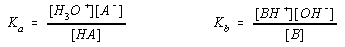We now understand why this is done. Because the concentration of water is so large, the reaction between an acid or a base and water is a pseudo-first-order reaction that only depends on the concentration of the acid or base.